#### You may also like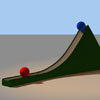### Whoosh

A ball whooshes down a slide and hits another ball which flies off the slide horizontally as a projectile. How far does it go?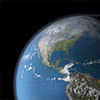### Escape from Planet Earth

How fast would you have to throw a ball upwards so that it would never land?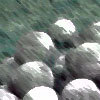### Cannon Balls

How high will a ball taking a million seconds to fall travel?

# Angle of Shot

##### Age 16 to 18Challenge Level
If a shotputter releases the shot at speed $v$, at angle $\theta$ to the ground and at a height $h$ above the ground, the trajectory of the shot is a parabola with coordinates determined by $(v\cos(\theta)t, v\sin(\theta)t - \frac{g}{2}t^2)$. Suppose the shot hits the ground at time T. Equating the $y$-component to $0$ gives $T^2 - \frac{2v\sin(\theta)}{g}T - \frac{2h}{g} = 0$. Solving gives $T=\frac{v\sin(\theta)}{g} + \sqrt{\frac{2h}{g} + \frac{v^2\sin^2(\theta)}{g^2}}$, so the range is therefore $d = \frac{v^2\sin(2\theta)}{2g}\left(1 + \sqrt{1 + \frac{2gh}{v^2\sin^2(\theta)}}\right)$

Assuming for the time being that the athlete can launch the shot at the same speed at any angle, I plotted a graph of range against projection angle for a variety of speeds: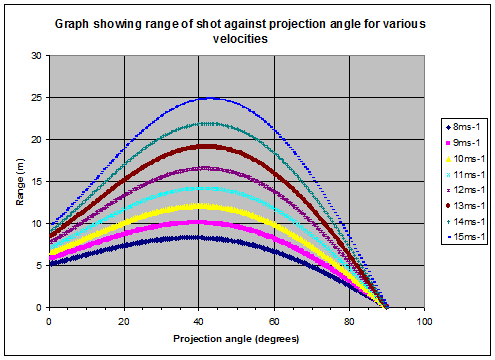Looking at the graph, it appears the optimal angle for maximising range is slightly smaller than $45^{\circ}$.

We now need to incorporate how the maximum launch speed varies with the projection angle. Here's a graph of projection velocity against projection angle. Although the projection velocity decreases as the projection angle increases, the range previously had a maximum at $45^{\circ}$, so the optimal angle will now be a tradeoff between angle and velocity.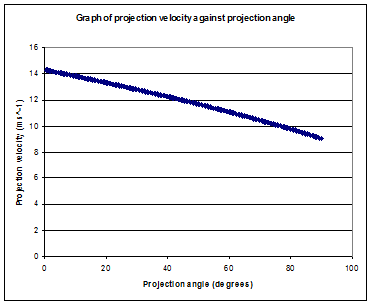We can now calculate the range of the shot using this projection velocity, and plot a graph of range against projection angle (using the same value of the parameters as before):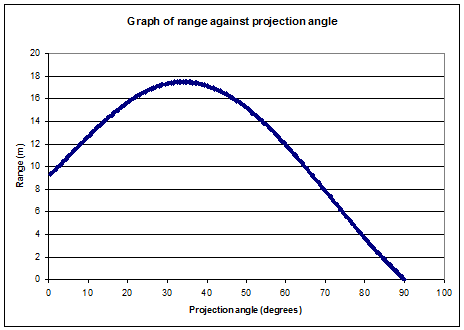For these values of the parameters,we can see the optimal angle is now around $35^{\circ}$, as opposed to $45^{\circ}$ in the previous model.

Solving this problem using differentiation is quite messy, but well done to Joshua who had a go; you can see what he did here.